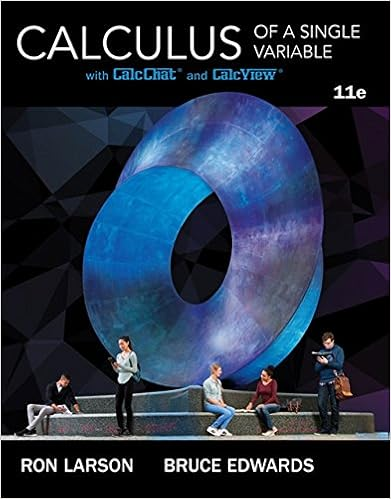a State the conclusion to the Change of Variables Theorem...

• Notes
• 7

This preview shows page 1 - 3 out of 7 pages.

We have textbook solutions for you!
The document you are viewing contains questions related to this textbook.The document you are viewing contains questions related to this textbook.
Chapter 6 / Exercise 2
Calculus of a Single Variable
Edwards/LarsonExpert Verified
Mock Exam 4 – Version 1. December 1, 2009 Tuesday This is longer than the actual 50-minute exam, but work on as many as possible on your own. The actual exam will have 6 to 8 problems, all of which you should complete. 1. (a) State the conclusion to the Change of Variables Theorem for Double Integrals and explain the role of the Jacobian. Why is there an absolute value around the Jacobian in the Change of Variables Theorem for Double Integrals? (b) Determine an appropriate change of variables and evaluate the integral ZZ D r x + y x - 2 y dA where D is the region in R 2 enclosed by the lines y = x/ 2, y = 0, and x + y = 1. 2. Express the integral Z 3 0 Z x 0 dydx p x 2 + y 2 as an iterated integral in polar coordinates. 3. Let W be the region in R 3 above the xy -plane bounded by the two spheres x 2 + y 2 + z 2 = 9 and x 2 + y 2 + z 2 = 25. Set up and then evaluate an iterated integral(s) that will calculate the volume of W . 4. [This is a review problem in order to help you distinguish scalar line integrals, vector line integrals, scalar surface integrals, and vector surface integrals.] Consider a metal wire in the first quadrant in the shape of the circle x 2 + y 2 = 4. (a) Find a parametrization of the wire. (b) The density at a point ( x, y ) on the wire is given by f ( x, y ) = 4 x - 3 y g/cm. Compute the mass of the wire. (c) If your parametrization in C was reoriented, how could that effect the integral computed in part (b)? Briefly explain your answer. (d) The integral in part (b) is called ca ar line integral. It computes m s, where f ( x, y ) = 4 x - 3 y is called a d n ty function. 5. (a) Compute Z C ydx - xdy x 2 + y 2 , where C is the circle of radius 1 centered at the origin, oriented counterclockwise. (b) State the formula at the conclusion of Green’s Theorem. (c) Would Green’s Theorem have been an appropriate alternative to evaluating the line integral in part (a)? Briefly explain your answer. (d) The integral in part (a) is called e or line integral. It computes w k. We say F ( x, y ) = ( , ) is a f ce field function. 6. (a) Let S be the sphere x 2 + y 2 + z 2 = 16. Parametrize the sphere. (b) Evaluate ZZ S ( x 2 + y 2 + z 2 ) dS . (c) The integral in part (b) is called ca ar surface integral. It computes m s, where f ( x, y, z ) = ( x 2 + y 2 + z 2 ) is called a d n ty function.
We have textbook solutions for you!
The document you are viewing contains questions related to this textbook.The document you are viewing contains questions related to this textbook.
Chapter 6 / Exercise 2
Calculus of a Single Variable
Edwards/LarsonExpert Verified
7. Let S be the part of the cylinder x 2 + z 2 = 4 bounded by - 1 / 2 y 1 / 2. (a) Find a parametrization of S . (b) Find the general formula for the normal vector. (c) Let F ( x, y, z ) = ( x, sin y, z ). Compute the flux of the vector field F across the surface S . (d) The integral in part (c) is called e or surface integral. It computes f x. We say F is a v tor field function. (e) If T is the part of the cylinder x 2 + z 2 = 4 bounded by the sphere x 2 + y 2 + z 2 = 1 / 4, what is the parametrization of T ? 8. (a) State the formula at the conclusion of Stokes’ Theorem. (b) Evaluate RR S ( ∇ × F ) · ndS , where F ( x, y, z ) = ( e z 2 , 4 x - y, 8 z sin y ) and where S is the portion of the paraboloid z = 4 - x 2 - y 2 above the xy -plane, oriented so that the unit normal vectors point to the outside of the paraboloid.
•••